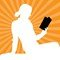# Why is 'X' used so commonly in maths equation?In algebric or any equation based mathematical problem the letter 'X' is mostly used as variable. You will rarely see any other letter as variable. Is there any specific reason why 'X' is used? Because it seems so random. If it was 'a' or something then it will be understandable as it is a very common letter but why 'X' only when it isn't really common (except maths)?
Why is 'X' used so commonly in maths equation?
23 Opinion